# Lending Ratios

These are utilized by banks and other lending organizations to analyze credit.Reviewed by

Expertise: Private Equity | Investment Banking

Updated:

September 17, 2022

Banks and other lending organizations utilize lending ratios, also known as qualifying ratios, to analyze credit. In addition, financial institutions conduct due diligence, which is a thorough investigation of the borrower's past and economic history, before assigning a credit score to a borrower.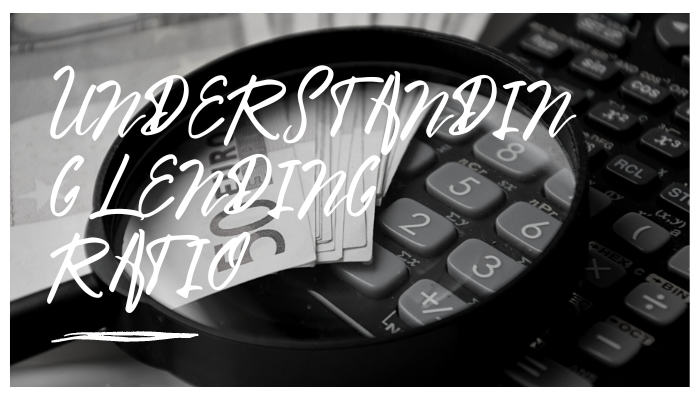When underwriting and approving loans, lending ratios are frequently used. Different lenders use lending ratios differently. Various ratios are used in credit analysis, and the decision is made based on the borrower's objectives and the projects for which they intend to use funds.

The ratios assist in determining whether private individuals or institutions can repay loans promptly. There are both qualitative and quantitative methodologies used in the credit analysis process. A crucial component of quantitative analysis is lending ratios.

• Before a loan is originated, potential borrowers are subjected to credit review.
• They consist of loan-to-value, housing expense, and debt-to-income ratios.
• Different lenders use lending ratios differently.

## Types

The qualifying ratio specifications may differ between lenders and loan programs. Therefore, they are frequently considered while reviewing an application along with the borrower's credit score.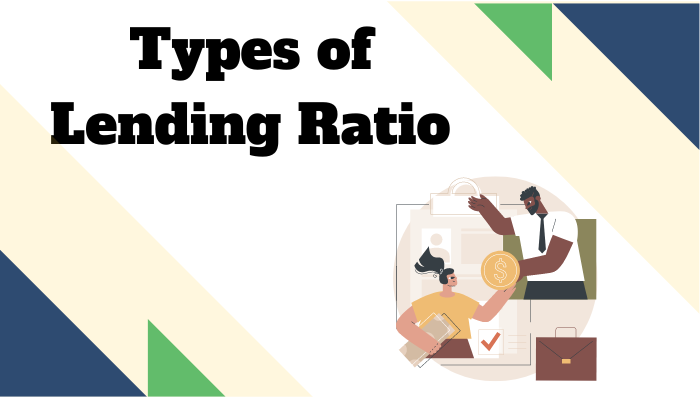Two of the most common and significant qualifying ratios in consumer financing are the debt-to-income ratio and the housing expense ratio. Therefore, the borrower's debt-to-income ratio will substantially consider specific credit products (personal loans, credit cards).

Mortgage financing will consider both the debt-to-income ratio and the housing expense ratio.

Below you will be able to see the various lending ratios that financial institutions may use to assign a credit score to individuals or other institutions, underwrite loans, or analyze credit.

## Debt-to-Income Ratio

The debt-to-income ratio (DTI) is a lending ratio that compares a person's monthly debt payments to their gross monthly income to act as a personal financial indicator.

Gross income is the amount received each month before expenses, like taxes, interest costs, etc.

Put another way, the debt-to-income ratio is the proportion of gross revenue used to pay debts (paying off debt). The balance is determined by dividing the monthly debt payments by the gross monthly income.

The formula is as follows:

Debt-to-Income Ratio = Total Monthly Debt Payments / Gross Monthly Income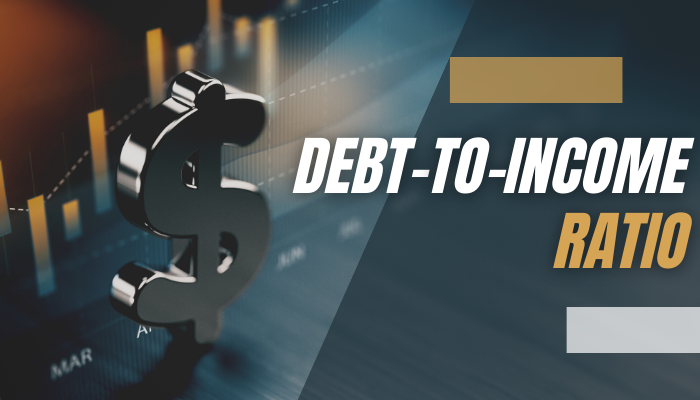When determining a person's capacity to make regular debt payments on a property acquired with borrowed money, mortgage lenders sometimes use the DTI ratio as a statistic.

How is the DTI ratio interpreted?

Lenders assess a person's financial status after calculating the DTI ratio using the person's gross monthly income and debt repayment costs.

A low debt-to-income ratio shows an acceptable balance between income and debt. A potential borrower's DTI ratio, for instance, of 14 percent indicates that 14 percent of their gross monthly income is used to pay off debt each month (debt service).

On the other hand, if the DTI ratio is relatively high, let's say it's 43 percent, which tells a lender that the borrower has a lot of debt relative to their monthly income. By the way, a borrower can only have a DTI ratio of 43 percent and still be approved for a loan.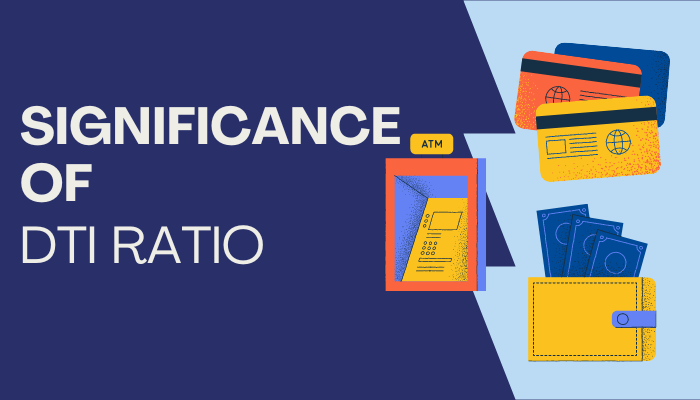So the lower the debt to income ratio, the more likely the borrower won't have any issues repaying the debt.

Therefore, before originating loans, banks and other credit providers prefer to see low DTI percentages for borrowers. Lenders typically desire a DTI ratio that is less than 36 percent.

## Housing Expense Ratio

A lending ratio called the housing expense ratio compares pre-tax income to housing expenses.

When evaluating the credit profile of a potential borrower, the ratio is frequently used in conjunction with the debt-to-income balance. The maximum amount of credit that can be extended to a borrower is also decided using this information.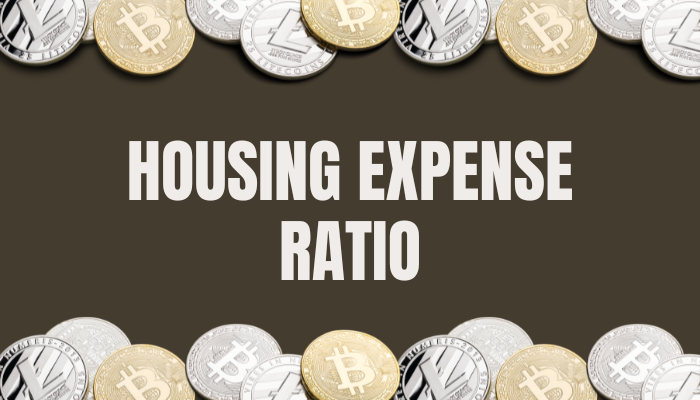Pre-tax income disclosure is necessary for the housing expense ratio since it affects both the debt-to-income ratio and the housing expense ratio.

A lender will total all a borrower's housing expense commitments to determine the housing expense ratio. They cover operational expenditures, including future mortgage principal and interest payments, property taxes and insurance, homeowner association dues, etc.

The housing expense ratio is calculated by adding up all expenses and dividing the total by the borrower's pre-tax income.

The formula is as follows:

Housing Expense Ratio = Housing Expenses / Pre-Tax Income

Notably, both annual and monthly payments can be used to compute the housing expense ratio. As a result, the housing expenditure ratio cutoff point established by lenders for approval of mortgage loans usually is equivalent to 28%.

## Loan-to-Value Ratio

Financial institutions utilize the loan-to-value ratio (LTV) to determine the lending risk before approving a mortgage to acquire real estate.A certain amount of an asset's value, such as the value of a house that will be issued as debt to a borrower, is represented by the loan-to-value ratio. Based on the borrower's credit score, the lender determines the funding portion after due diligence.

For instance, a bank will first issue a credit score to a person if they want to buy a property valued at $100,000 and want to get financing for it. Then, the credit evaluation will determine the maximum mortgage amount that can be given to purchasing a home. The formula is as follows: Loan-to-Value Ratio = Amount of Mortgage / Property Value Say the lender receives a$70,000 loan from the bank. As determined by the calculation above, the LTV will be 70% ($70,000 /$100,000). This means the borrower would be responsible for paying the final $30,000 of the property's worth.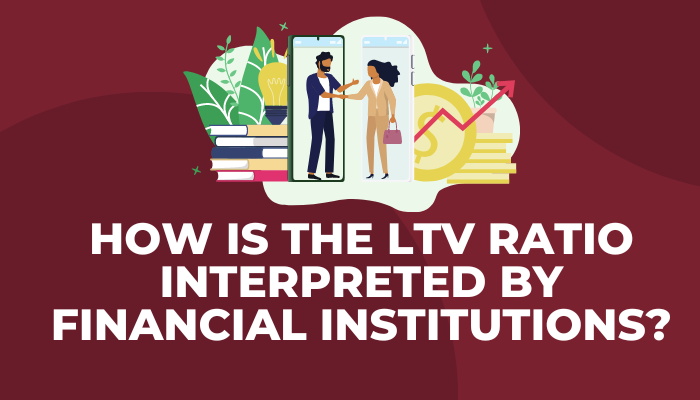How do financial institutions interpret the LTV ratio? The danger increases as the loan-to-value ratio increases. This is because the return (compensation) a lender will demand in exchange for the loan issuance will increase in direct proportion to the risk. Therefore, borrowers with more excellent LTV ratios than those with lower LTV ratios will have to pay higher interest rates. ## Working Capital Ratio The working capital ratio, commonly referred to as the current ratio, shows the proportion of existing assets to current liabilities that a business has.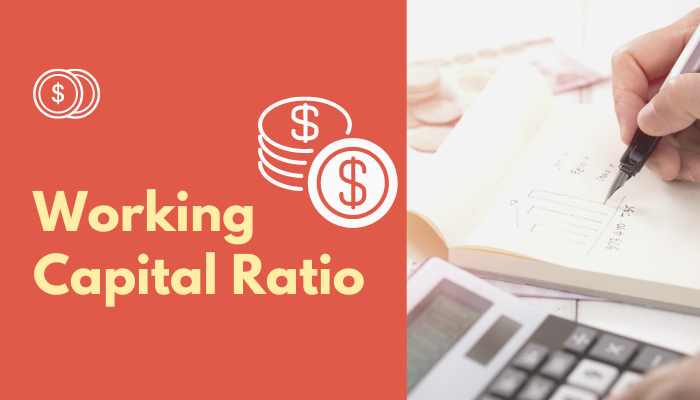The ratio demonstrates how quickly the company can pay its due short-term debts in less than a year. Therefore, current assets divided by current liabilities is the working capital ratio. The formula is as follows: Working Capital Ratio = Current Assets / Current Liabilities • On the current asset side, the principal accounts that will be shown here are: • The accounting for current liabilities is as follows: • Accounts payable • Short-term debt • Current portion of long-term debt • Interpreting the working capital ratio Suppose the company's working capital ratio is more significant than one. In that case, it is clear it has more current assets than liabilities and can, therefore, pay all of its existing commitments within the year using its current assets alone. ## Debt Service Coverage Ratio The debt service coverage ratio, or DSCR, gauges a company's capacity to use the operating income to pay off its debt. To put it another way, the DSCR shows how readily a business can pay down its debt using operating income. The following are the many methods for calculating the DSCR: • DSCR = EBITDA / Interest + Principal • DSCR = (EBITDA – Capital Expenditure) / Interest + Principal • DSCR = EBIT / Interest + Principal Debt Service Coverage Ratio InterpretationA lower ratio indicates a higher likelihood of default. If the DSCR is less than 1, the business may be unable to pay its debts off with just operating income. It pays principal and interest payments that are more than operating profit, EBITDA less: capital expenditures, or any other numerator used in the formula. Generally, a positive indicator is shown across all industries if the DSCR is greater than 2. For instance, the DSCR must be extraordinarily high—on average, seven times—in the coal mining sector. It is costly because coal mining is highly cyclical and necessitates a lot of capital investments. Therefore, lenders need to exercise extreme caution when making loans to coal mining enterprises. The service sector, however, only needs a DSCR of three and a half times. Therefore, the capital expenditures and cyclicality in the service sector are pretty low. ## Debt-to-Equity Ratio The debt-to-equity (D/E) ratio, which measures a company's financial leverage, is determined by dividing all of its obligations by its shareholders' value. An essential statistic in corporate finance is the D/E ratio.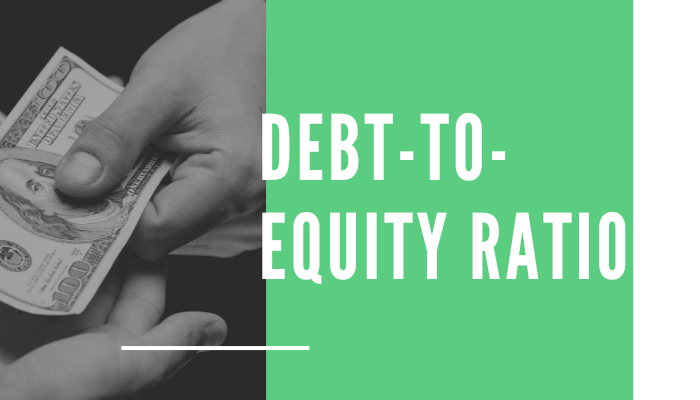It gauges how much debt a business uses to finance operations instead of owned cash. More specifically, it shows whether shareholder equity would be sufficient to pay off all debts in the event of a downturn in business. A specific kind of gearing ratio is the debt-to-equity ratio. The debt-to-equity ratio highlights a company's capital structure. The equation is: Debt-to-Equity Ratio = Total Liabilities / Shareholders’ Equity Long-term and short-term debt, along with any additional liabilities, make up total liabilities. Another option is to use the more cautious formula: Total Liabilities-to-Tangible Net Worth Ratio = Total Liabilities / (Shareholders' Equity – Intangible Assets) Using the Debt-to-Equity Ratio to Interpret. A high debt-to-equity ratio frequently indicates an aggressive growth strategy. Conversely, insolvency may ensue if the additional debt does not generate more revenue. ## The lending ratio for banks Whether or not to lend money is made in a manner comparable to that of commercial banks and is contingent on gaining clearance from a risk section. This division is responsible for calculating the losses the bank may suffer due to the exposure under consideration.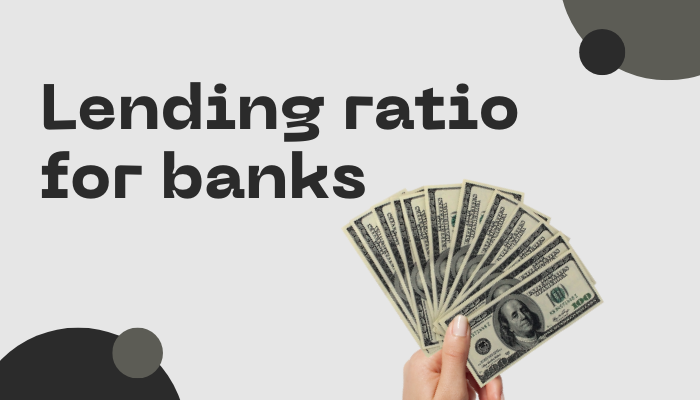The bank needs to ensure that it is generating a good return on its capital; therefore, each loan must not only pass a test of investment or lending return, but this is also a requirement for the loan. Three fundamental lending ratios are applied in the process of calculating returns. These ratios are the return on assets, the return on equity, and the return on risk-weighted assets. ## How a corporate bank evaluates a loan The steps are as follows: Step 1: Calculate net interest margin Finding loan level net income is the first stage in the loan evaluation process. The formula for calculating the NIM: Interest income from the loan plus other fees and charges, fewer interest costs (the cost of funds from customer deposits or borrowing money from elsewhere). Step 2: Calculate loan level net income. After that, you deduct an expense known as a loan loss provision, a contingency or condition, representing the estimated amount of money that must be set aside for any anticipated losses on the loan. Finally, you must deduct taxes, allocated overhead, and related personnel costs/salaries. This will raise the corporate bank's net income to loan level levels. Step 3: Determine Key Lending Ratios Using a return on assets ratio, the loan level net income is compared to the total loan amount (keep in mind that the loan is an asset for the bank): Return on Assets (ROA) = Net income / total assets a) Knowledge of Return on Assets To determine whether or not to extend a loan to a potential borrower, ROA is utilized to determine how profitable a likely loan is.The banks can authorize the loan if other conditions are met from a risk viewpoint, such as exposure to the same client, exposure to the industry at large, and anticipated losses from the loan if these profitability estimates or ratios pass the bar for the lending product. It should be noted that return on assets is not directly comparable across lending products; therefore, if product A is less risky than product B, a ROA of 1% is not necessarily worse than a ROA of 3%. Therefore, the decision regarding the loan evaluation depends on various barriers for various products. ROA has its limitations because different items differ from one another. And utilizing a return on risk-weighted assets ratio to risk-adjust the loan amounts is one method of adjusting for the variations in risks across products. b) Recognizing RORWA Return on Risk Weighted Assets (RORWA) = Net income / risk-weighted assets To account for the risks taken to generate profits, RORWA adjusts ROA. A$25 million loan with a $1 million net profit, for instance, would have a ROA of 4%.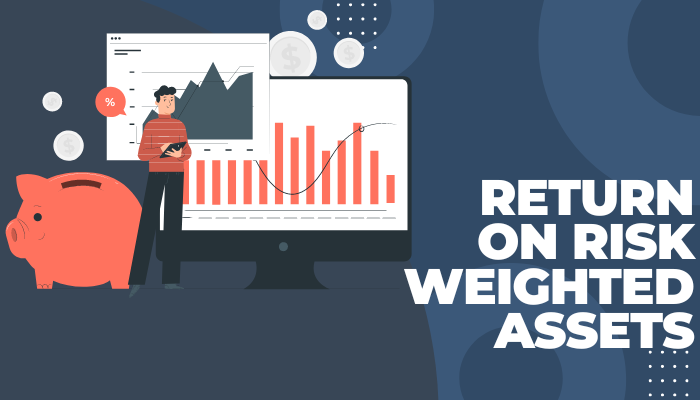However, if the loan was considered safe, the risk weighting factor (RORWA) may be reduced from$25 million to $20 million, or even to 5%. RORWA is a valuable technique to standardize the corporate bank's assessment of the loan's profitability. While RoRWA adjusts the number of assets based on their perceived risk, ROA employs assets as the denominator in the formula. Take note that the numerator could represent pre-tax profit. c) Recognizing ROE Return on equity = Net income / Equity When a bank issues a loan, it transfers its safest asset—cash—to the borrower, establishing a new (riskier) support. Bank balance sheets will become increasingly difficult if this is allowed to continue, putting deposits at risk.Regulators mandate that banks assign a specific amount of capital at risk depending on the credit profiles of a potential borrower that their risk assessment models recommend protecting depositors. Consider a$25 million loan with a $1 million annual income that also demands a$5 million equity reserve from the bank. Therefore, the ROE is $1,000,000 /$5,000,000 = 20%.

Step 4: Adjust to reflect investment banking relationships

Corporate Banking, which is part of the Investment Bank, as we mentioned in our Ultimate Guide to Corporate Banking, will occasionally approve loans that wouldn't be profitable on their own but make sense because they allow other departments of the Investment Bank to offer follow-on services like M&A, debt and equity underwriting, etc.

### Everything You Need To Build Your RE Modeling Skills

To Help You Thrive in the Most Rigorous RE Interviews and Jobs.

Researched and authored by Ruxue Bai | LinkedIn